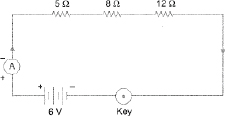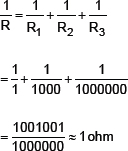# Important Questions for Board Exam:ElectricityImportant Questions, Revision Notes and Video Lectures on Real Numbers for Class 10 Board Exam (Padhte Chalo, Badhte Chalo- Eckovation). Join Group Code 101010 to discuss your doubts with educators from IIT Delhi

• Electricity comes under IV unit which includes Electricity and Magnetic Effects Of Current
• This unit carries 13 marks out of 80Q1.  What does an electric circuit mean?
Ans. A continuous conducting path consisting of wires and other resistances and a switch between the two terminals of a cell or a battery along which an electric current flow is called a circuit.
Q2.  Define the unit of current.
Ans. The unit of current is Ampere. 1 Ampere is said to be when 1 coulomb of charge flow through any cross section of a conductor in 1 second.
Q3.  Calculate the number of electrons constituting one coulomb of charge.
Ans. 1C = 6 × 1018 electrons.
Q4.  Name a device that helps to maintain a potential difference across a conductor.
Ans. A battery of many cells or a single cell can maintain potential difference.
Q5.  What is meant by saying that the potential difference between two points is 1 V?
Ans. The potential difference between two points is said to be 1 V if 1 Joule of work is done in moving 1 Coulomb of charge from one point to another point
Q7.  How much energy is given to each coulomb of charge passing through a 6 V battery?
Ans. Amount of charge = 1 coulomb or 1C
Potential difference = 6 V
Energy or work done = ?
Work done or energy = V × Q[Q is the amount of charge flowing between two points at potential difference V = 6
i.e., W = V × Q
W = 6 × 1
Work done or energy = 6 joules

Q8.  On what factors do the resistance of a conductor depend?
Ans. Resistance of a conductor depends on:

(a)  Length of a conductor. Resistance of a conductor is directly proportional to the length of a conductor. If length increases resistance will also increase.
(b)  Area of cross section of a conductor. The resistance of a conductor is inversely proportional to the area of the cross section of a conductor.
(c)  Effect of material of a conductor. The resistance of a conductor also depends on the material of a conductor.
E.g. The resistance of nichrome wire is 60 times more than that of copper wire as nichrome has high electrical resistance
(d)  Effect of temperature. The resistance of all pure metals increases on increasing the temperature and decreases on decreasing the temperature.
Q9.  Will current flow more easily through a thick wire or a thin wire of the same material, when connected to the same source? Why?
Ans. The current will flow more easily through a thick wire as compared to the thin wire because the resistance of thick wire is less than that of thin wire. Less resistance, means more current.
Q10.  Let the resistance of an electrical component remains constant while the potential difference across the two ends of the component decreases to half of Us fanner value. What change will occur in the current through it?
Ans. Amount of current will be halved.
Q11.  Why are coils of electric toasters and electric irons made of an alloy rather than a pure metal?
Ans. The coils of such heating appliances are made up of an alloy rather than a pure metal because:
(a)  The resistivity of an alloy is much higher than that of a pure metal.
(b)  An alloy does not undergo oxidation easily even at high temperature when it is even red hot.
Q12  (a) Which among iron and mercury is a better conductor?
(b)  Which material is the best conductor?
Ans. (a)  Iron
(b)  Silver

Q13  Draw a schemata: diagram of a circuit consisting of a battery of three cells of 2 V each, a 5 Ω LI resistor, 8 Ω resistor, and a 12 Ω resistor, and a plug key, alt connected in seriess.

Ans.Q14.  Redraw the circuit of Question 12, putting in an ammeter to measure the current through the resistors and a voltmeter to measure the potential different across the 12 Ω resistors. What would be the readings in the ammeter and the voltmeter?Rs = 5 Ω + 8 Ω + 12 Ω = 25 Ω
V = 6 V
I = ?= 0.24 A
When R = 12 Ω
V = IR
= 0.24 x 12
= 2.88 V
Hence, reading in the ammeter would be 0.24 A and reading in voltmeter would be 2.88 V
Q15.  Judge the equivalent resistance when Die following are connected in parallel—
(a) 1 Ω , 106 Ω , (b) 1 Ω , 103 Ω and 106 Ω.
Ans. (a)  1 ohm and 106 times ohm
R1 = 1 ohm
R2 = 106 times = 1000000 ohm
Total resistance in parallel 1/R = 1/R1 + 1/R2
= 1/1 + 1/1000000
= 1000000+1/1000000
= 1000001/1000000
= 1/R = 1/1 ohm (approx)
Resistance = 1 ohm (approx)
(b)  R1 = 1 ohm
R2 = 103 ohm
R3 = 106 ohm
Total resistance in parallelQ16.  An electric lamp of 100 Ω, a toaster of resistance. 50 Ω , and a water filter of resistance 500 Ω are connected in parallel to a 220 V source. What is the resistance of an electric iron connected to the same source that takes as much current as all the three appliances, and what is the current through it?
Ans. Resistance of electric lamp,toaster & water filter=100 ohm, 50 ohm & 500 ohm Respectively
Potential difference = 220 volt
Total resistance of the three appliances in parallel = 1/R = 1/R1 + 1/R2 + 1/R3
= 1/100 + 1/50 + 1/500
1/R = 5 + 10 + 1/500
1/R = 16/500 = 4/125
= 125/4 = 31.25 ohm
V = I × R
220 = I × 31.25
I = 7.04 amp
Hence current through the electric iron = 7.04 amp.
Q17.  What are the advantages of connecting electrical devices in parallel with the battery instead of connecting them in series?
Ans. Advantages of parallel connection are:
(a)  In parallel circuit, if one electric appliance stop working due to some defect, then all other appliances keep working normally.
(b)  In parallel circuit, each electrical appliance has its own switch due to which they can be turned on or off without affecting other appliances.
(c)  Each electrical appliance get the same voltage (220 V) as that of the power supply line.
(d)  In parallel connection of electrical appliances, the overall resistance of the circuit is reduced due to which the current from the power supply is high.
Q18.  How can three resistors of resistances 2 Ω , 3 Ω , and 6 Ω be connected to give a total resistance of (a) 4 Ω , (b) 1 Ω?
Ans. R1 = 2 ohm
R2 = 3 ohm
R3 = 6 ohm
(a)  When R2 and R3 are connected in parallel with R1 in series we get
1/R = 1/R2 + 1/R3
g= 1/3 + 1/6
= 1/2
Thus, R = 2 ohm
Resistance in series = R + R1
= 2 + 2
= 4 ohm
(b)  When R1,R2, R3 are connected in parallel we get
1/R = 1/R1 + 1/R2 + 1/R3
= 1/2 + 1/3 + 1/6
= 1 ohm.
Q19.  What is (a) the highest, (b) the lowest total resistance that can be secured by combinations of four coils of resistance 4 Ω , 8 Ω , 12 Ω , 24 Ω?
Ans. (a) The highest resistance is when the resistances are connected in series:
R1 = 4 ohm                R2 = 8 ohm
R3 = 12 ohm                R4 = 24 ohm
Total resistance in series = R1 + R2 + R3 + R4
= 4 + 8 + 12 + 24
= 48 ohm
Thus, highest resistance is 48 ohm.
(b)  The lowest resistance is when the resistances are connected in parallel
Total resistance in parallel
= 1/R1 + 1/R2 + 1/R3 + 1/R4
1/R = 1/2 + 1/8 + 1/12 + 1/24
= 12/24
1/R = 1/2 ohm, R = 2 ohm
Thus, lowest resistance is 2 ohm.
Q20.  Why does the cord of an electric heater no, glow while the heating element does?
Ans. The resistance of cord is extremely small as compared to that of heating element, so the heat produced in cord is less as compared to heating element. So the heating element begins to glow but cord does not glow